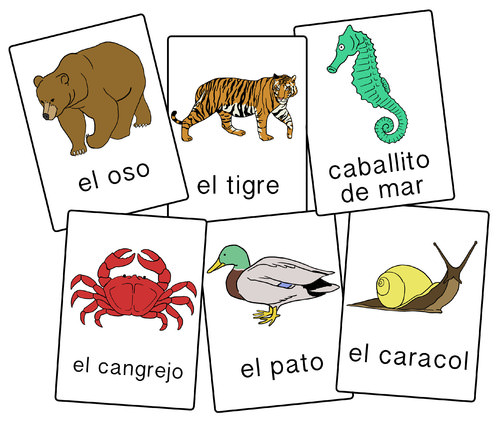# 6.2: Mendeleev's Periodic Table

$$\newcommand{\vecs}{\overset { \rightharpoonup} {\mathbf{#1}} }$$ $$\newcommand{\vecd}{\overset{-\!-\!\rightharpoonup}{\vphantom{a}\smash {#1}}}$$$$\newcommand{\id}{\mathrm{id}}$$ $$\newcommand{\Span}{\mathrm{span}}$$ $$\newcommand{\kernel}{\mathrm{null}\,}$$ $$\newcommand{\range}{\mathrm{range}\,}$$ $$\newcommand{\RealPart}{\mathrm{Re}}$$ $$\newcommand{\ImaginaryPart}{\mathrm{Im}}$$ $$\newcommand{\Argument}{\mathrm{Arg}}$$ $$\newcommand{\norm}{\| #1 \|}$$ $$\newcommand{\inner}{\langle #1, #2 \rangle}$$ $$\newcommand{\Span}{\mathrm{span}}$$ $$\newcommand{\id}{\mathrm{id}}$$ $$\newcommand{\Span}{\mathrm{span}}$$ $$\newcommand{\kernel}{\mathrm{null}\,}$$ $$\newcommand{\range}{\mathrm{range}\,}$$ $$\newcommand{\RealPart}{\mathrm{Re}}$$ $$\newcommand{\ImaginaryPart}{\mathrm{Im}}$$ $$\newcommand{\Argument}{\mathrm{Arg}}$$ $$\newcommand{\norm}{\| #1 \|}$$ $$\newcommand{\inner}{\langle #1, #2 \rangle}$$ $$\newcommand{\Span}{\mathrm{span}}$$Figure $$\PageIndex{1}$$ (Credit: Laura Guerin; Source: CK-12 Foundation; License: CC BY-NC 3.0)

### When you study for a test, how do you approach the task?

One useful way to study for a test is to use flashcards. To make them, write down vocabulary words, foreign language terms, math formulas, chemistry reactions—anything you want to learn. Then sort these cards into categories and topics that go together. This organization of information helps you see patterns in the material so that you can tie different ideas together and make better sense of them.

The periodic table was first built using a set of cards. With this strategy, Dmitri Mendeleev, the creator of the periodic table, could organize and rearrange material until patterns emerged.

## Mendeleev's Periodic Table

In 1869, Russian chemist and teacher Dmitri Mendeleev (1836-1907) published a periodic table of the elements. The following year, German chemist Lothar Meyer independently published a very similar table. Mendeleev is generally given more credit than Meyer because his table was published first, and because of several key insights that he made regarding the table.Figure $$\PageIndex{2}$$: Dmitri Mendeleev. (Credit: Unknown; Source: http://commons.wikimedia.org/wiki/File:DIMendeleevCab.jpg; License: Public Domain)

Mendeleev was writing a chemistry textbook for his students and wanted to organize all of the known elements at that time according to their chemical properties. He famously organized the information for each element onto separate note cards that were then easy to rearrange as needed. He discovered that when he placed them in order of increasing atomic mass, certain similarities in chemical behavior repeated at regular intervals. This type of a repeating pattern is called "periodic". A pendulum that swings back and forth in a given time interval is periodic, as is the movement of the moon around the Earth.Figure $$\PageIndex{3}$$: Mendeleev's 1869 periodic table. (Credit: Dmitri Mendeleev; Source: http://commons.wikimedia.org/wiki/File:Mendeleev%2527s_1869_periodic_table.png; License: Public Domain)

In the figure above, atomic mass increases from top to bottom of vertical columns, with successive columns going left to right. As a result, elements that are in the same horizontal row are groups of elements that were known to exhibit similar chemical properties. One of Mendeleev's insights is illustrated by the elements tellurium $$\left( \ce{Te} \right)$$ and iodine $$\left( \ce{I} \right)$$. Notice that tellurium is listed before iodine even though its atomic mass is higher. Mendeleev reversed the order because he knew that the properties of iodine were much more similar to those of fluorine $$\left( \ce{F} \right)$$, chlorine $$\left( \ce{Cl} \right)$$, and bromine $$\left( \ce{Br} \right)$$ than they were to oxygen $$\left( \ce{O} \right)$$, sulfur $$\left( \ce{S} \right)$$, and selenium $$\left( \ce{Se} \right)$$. He simply assumed that there was an error in the determination of one or both of the atomic masses. This turned out not to be the case, but Mendeleev was indeed correct to group these two elements as he did.

Notice that there are several places in the table that have no chemical symbol, but are instead labeled with a question mark. Between zinc $$\left( {Zn} \right)$$ and arsenic $$\left( \ce{As} \right)$$ are two such missing elements. Mendeleev believed that elements with atomic masses of 68 and 70 would eventually be discovered and that they would fit chemically into each of those spaces. Listed in the table below are other properties that Mendeleev predicted for the first of these two missing elements, which he called "eka-aluminum", compared with the element gallium.

 Eka-Aluminum $$\left( \ce{Ea} \right)$$ Gallium $$\left( \ce{Ga} \right)$$ Atomic Mass $$68 \: \text{amu}$$ $$69.9 \: \text{amu}$$ Melting Point Low $$30.15^\text{o} \text{C}$$ Density $$5.9 \: \text{g/cm}^3$$ $$5.94 \: \text{g/cm}^3$$ Formula of Oxide $$\ce{Ea_2O_3}$$ $$\ce{Ga_2O_3}$$

The element gallium was discovered four years after the publication of Mendeleev's table, and its properties matched up remarkably well with eka-aluminum, fitting into the table exactly where he had predicted. This was also the case with the element that followed gallium, which was eventually named germanium.

Mendeleev's periodic table gained wide acceptance with the scientific community and earned him credit as the discoverer of the periodic law. Element number 101, synthesized in 1955, is named mendelevium after the founder of the periodic table. It was, however, several years after Mendeleev died before the several discrepancies with the atomic masses could be explained, and before the reasons behind the repetition of chemical properties could be fully explained.

## Summary

• Mendeleev published his periodic table in 1869.
• His organization of elements was based on atomic mass.
• Mendeleev's periodic table made it possible to predict properties of elements that had not yet been discovered.

## Review

1. When did Mendeleev publish his periodic table?
2. Who else came out with a periodic table at about the same time?
3. Why was Mendeleev’s table considered to be superior?
4. What element did Mendeleev predict to exist?
5. What element was named after Mendeleev?

6.2: Mendeleev's Periodic Table is shared under a CK-12 license and was authored, remixed, and/or curated by CK-12 Foundation via source content that was edited to conform to the style and standards of the LibreTexts platform; a detailed edit history is available upon request.